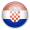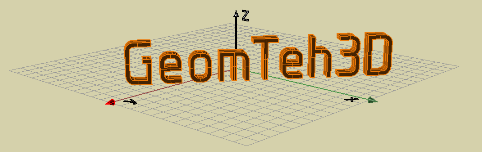Developing project of University of Zagreb

 Plane Geometry Educational materials for lectures and exercises containing interactive files supported by GeoGebra. Extended Euclidean Plane Second Order Curves - Conic Sections Introduction to Algebraic Curves Classification of Conics, Order and Class of Conics Conjugate Diameters of Conics On Curvature of Conics Construction of Second Order Curves Ellipse Parabola Hyperbola Transformations of the Plane Introduction to Collineations Perspective Collineation Basic Properties and the Perspective Collinear Image of a Point Perspective Collinear Image of a Straight Line Vanishing Lines Perspective Collinear Image of a Line Segment Collinear Image of Polygons Perspective Collinear Image of a Circle Affine Transformation Basic Properties and Affine Image of a Point Affine Image of a Straight Line Affine Image of a Line Segment Affine Image of Polygons Affine Image of a Circle
 Space Geometry – Method of Projections Educational materials for lectures and exercises containing visualizations and animations supported by Mathematica and interactive files supported by GeoGebra and PowerPoint. Extended Euclidean Space Basic Geometrical Relationships Solids Monge's Method of Projections (or Orthogonal Projections) Introduction to Monge's Method of Projections (or Orthogonal Projections) Orthogonal Projection of a Point Orthogonal Projection of a Straight Line Orthogonal Projection of a Line Segment and its Length Orthogonal Projection of a Solid with a Base Parallel to the Plane of Projection Profile Projection Orthogonal Projection of a Plane Point of Intersection of a Line and a Plane Orthogonality Orthogonal Projection of Plane Figures and Rotation Orthogonal projection of solids in arbitrary position. PREPAERING MATERIALS FOR EXERCISES SOME SOLVED EXERCISES (drawings supported by Rhino) Axonometry Elevated Projection
 Space Geometry 3D Computer Modeling Educational materials for lectures, exercises and independently learning. It contains visualizations and animations supported by Mathematica, and about 50 five minutes long videos helping to overcome basics of Rhino 3D modeling. Introduction to Software Rhinoceros (Rhino) Solid Modeling Supported by Rhino Surfaces Introduction to Surfaces and Planes Tangent Algebraic Surfaces Cones and Cylinders Quadric Surfaces – Second Order Algebraic Surfaces (ellipsoid, hyperboloid, paraboloid, cone, cylinder) Intersections of Surfaces with Planes Intersections of Second Order Cones Intersections of Second Order Cylinders Intersections of Sphere Architectural Dome SOLVED EXAMPLES SUPPORTED BY RHINO Intersections of Surfaces Space Curves Intersection Curves from Cones, Cylinders and Spheres Intersection of Two Cones Intersection of a Cone and a Cylinder Intersection of Two Cylinders Vaults Intersection by Sphere SOLVED EXAMPLES SUPPORTED BY RHINO Terrains Horizontal Road on Ideal Terrain ( Rhino) Straight Inclined Road on Ideal Terrain (Rhino) Topographical Surface (Rhino) Road on Terrain (Rhino) Layer (Rhino)
 Linear Perspective Educational materials for lectures and exercises containing Mathematica visualizations and interactive files supported by GeoGebra. Basic Properties Some Tasks Related to the Horizontal Plane Connection between Orthogonal Projection and Perspective Selected Examples Point of Intersection of a Straight Line and a Plane Plane Determined by a Straight Line and a Point not Laying on the Line Perspective of a Straight Line Determined by Two Points Perspective of a Circle Ellipse as a Perspective of a Circle Ellipse as a Perspective of a Circle Construction of Perspective from Two Scales